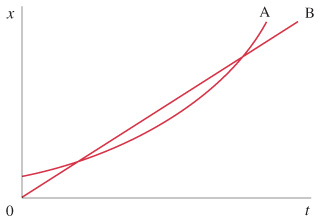Physics Practice Problems Velocity & Acceleration Graphs Practice Problems Solution: The figure shows the position vs. time graph for t...

⚠️Our tutors found the solution shown to be helpful for the problem you're searching for. We don't have the exact solution yet.

# Solution: The figure shows the position vs. time graph for two bicycles, A and B.Is there any instant at which the two bicycles have the same velocity?Which bicycle has the larger acceleration?At which instant are the bicycles passing each other?Which bicycle has the higher average velocity?

###### Problem

The figure shows the position vs. time graph for two bicycles, A and B.

Is there any instant at which the two bicycles have the same velocity?Which bicycle has the larger acceleration?

At which instant are the bicycles passing each other?

Which bicycle has the higher average velocity?

Velocity & Acceleration Graphs

Velocity & Acceleration Graphs

#### Q. The figure shows the velocity of a train as a function of time. (a) At what time was its velocity greatest?(b) During what periods, if any, was the ve...

Solved • Mon Oct 29 2018 19:00:11 GMT-0400 (EDT)

Velocity & Acceleration Graphs

#### Q. The graph in the figure describes the acceleration as a function of time for a stone rolling down a hill starting from rest. (a) Find the change in th...

Solved • Mon Oct 29 2018 18:50:20 GMT-0400 (EDT)

Velocity & Acceleration Graphs

#### Q. High-speed motion pictures ( 3500 ) of a jumping, 210 mu { m{g}} flea yielded the data used to plot the graph given in the figure . (See "The Flying L...

Solved • Mon Oct 29 2018 18:49:58 GMT-0400 (EDT)

Velocity & Acceleration Graphs

#### Q. A cat walks in a straight line, which we shall call the x-axis with the positive direction to the right. As an observant physicist, you make measureme...

Solved • Mon Oct 29 2018 18:49:57 GMT-0400 (EDT)